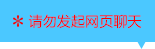| | |

||
APP端下载AndroidiPhone
￥160

|
1547人点赞
605814人已学习
|

3天无理?#36175;?#27454;

3天无理?#36175;?#27454;?#21644;?#27454;将以超级币形式退至您的超级课堂学习账户，便于您重新选购其他课程。恶意退款将被冻结账号。

• 1、函数奇偶性的概念，记住定义式。奇函数：$f(-x)=-f(x)$；偶函数：$f(-x)=f(x)$
2、 判断奇偶性的方法：(1)、判断定义域是否关于原点对称。(2)、求$f(-x)$
3、 奇偶函数组合的规律
• 1、奇偶函数各自的图像特征：偶函数的图象是关于$y$轴的轴对称图形
2、 奇函数的图象是关于原点的中心对称图形
3、 图像对称性和函数奇偶性的互推技巧
4、 利用图像求不等式解集
• 1、左右和上下平移后，函数对称轴或对称中心，以及奇偶性的变化规律
2、 牢记奇偶函数的对称中心和对称轴，再牢记?#30333;?#21152;右减，上加?#24405;酢?#30340;平移规律
• 1、由奇偶性和解析式求未知参数
2、 对于简单的解析式，就直接采用定义式。对于用定义式难以解决的题目，两大技巧：多项式函数法和原点法
3、 多项式函数法利用的就是组合函数奇偶性的规律，而原点法利用的就是特殊值法
• 1、对于求对称区间解析式的这类题，首先都是要求$f(-x)$，然后利用奇偶函数的定义式，得到$f(x)$的解析式
2、 对于求函数值的题目，直接利用定义式即可，注意奇偶函数对称区间值域的性质，灵活运用它就可以避免去计算解析式
• 1、一类特殊的函数，它由一个奇函数和一个常数项相加而成。即$g(x)=f(x)+c$，$f(x)$为奇函数。此时，它满足$g(x)+g(-x)=2c$，即当这种函数的自变量取相反数时，它们函数值的和刚?#26790;?#20108;倍的常数项
2、 这个结论也能反着用，即若已知$f(x)+f(-x)=2c$，则可知$f(x)$能写成奇函数+常数的形式
3、 一个诡异的扩展结论：若$f(x)+f(-x)=2c$，则$f(x)$的对称中心为$(0,c)$
• 1、已知$f(x+y)$与$f(x)$、$f(y)$的关系，可以将$-x$赋给$y$，这样就知道了$f(x)$、$f(-x)$与$f(0)$的关系。然后再赋值，求$f(0)$的大小，从而得到$f(-x)$与$f(x)$的关系，即可判断$f(x)$的奇偶性了
2、 已知$f(xy)$与$f(x)$、$f(y)$的关系，可以将$-1$赋给$y$，这样就知道了$f(-x)$、$f(x)$与$f(-1)$的关系。然后再赋值，求$f(-1)$，从而得到$f(-x)$与$f(x)$的关系，即可判断$f(x)$的奇偶性了
3、 判断抽象函数单调性，如果已知$f(xy)$与$f(x)$、$f(y)$的加减关系，要转化成差的形式，即$f(xy)-f(y)=f(x)$，从而用赋值法变成$f(x_{2})-f(x_{1})$；如果已知$f(xy)$与$f(x)$、$f(y)$的乘除关系，要转化成商的形式，注意在用作商法的时候，要证明$f(x)$在定义域内恒大于$0$
• 1、抽象函数的奇偶性和单调性的混合题型
2、 简单的函数值大小比较问题，用转化函数值法或图象分析法即可
3、 奇偶性和单调性之间存在一种很简单的规律即偶函数在其对称区间上的单调性相反；奇函数在其对称区间上的单调性相同
4、 $f(a)+f(b)＞0$或$＜0$

• 1• 2天使羽翼
• 3超级学员392904
• 4超级课堂现在有优惠政策加微信详细咨询18667177221
• 51
• 6超级学员896923
• 7超级学员947894
• 8超级学员956844
• 9随便看看
• 10超人强661329317人在学
￥ 240 ￥ 0607002人在学
￥ 240 ￥ 1981304776人在学
￥ 240 ￥ 0606991人在学
￥ 300 ￥ 198

• 0
• 点击分享有?#32654;?/div>
••app端下载关注微信号pk10开奖记录直播